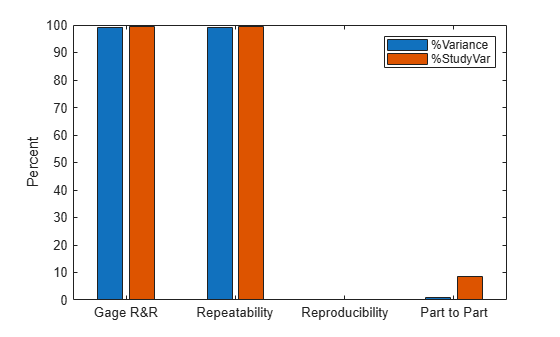# gagerr

Gage repeatability and reproducibility study

## Syntax

```gagerr(y,{part,operator}) gagerr(y,GROUP) gagerr(y,part) gagerr(...,param1,val1,param2,val2,...) [TABLE, stats] = gagerr(...) ```

## Description

`gagerr(y,{part,operator})` performs a gage repeatability and reproducibility study on measurements in `y` collected by `operator` on `part`. `y` is a column vector containing the measurements on different parts. `part` and `operator` are categorical variables, numeric vectors, character matrices, string arrays, or cell arrays of character vectors. The number of elements in `part` and `operator` should be the same as in `y`.

`gagerr` prints a table in the command window in which the decomposition of variance, standard deviation, study var (5.15 `x` standard deviation) are listed with respective percentages for different sources. Summary statistics are printed below the table giving the number of distinct categories (NDC) and the percentage of Gage R&R of total variations (PRR).

`gagerr` also plots a bar graph showing the percentage of different components of variations. Gage R&R, repeatability, reproducibility, and part-to-part variations are plotted as four vertical bars. Variance and study var are plotted as two groups.

To determine the capability of a measurement system using NDC, use the following guidelines:

• If NDC > 5, the measurement system is capable.

• If NDC < 2, the measurement system is not capable.

• Otherwise, the measurement system may be acceptable.

To determine the capability of a measurement system using PRR, use the following guidelines:

• If PRR < 10%, the measurement system is capable.

• If PRR > 30%, the measurement system is not capable.

• Otherwise, the measurement system may be acceptable.

`gagerr(y,GROUP)` performs a gage R&R study on measurements in `y` with `part` and `operator` represented in `GROUP`. `GROUP` is a numeric matrix whose first and second columns specify different parts and operators, respectively. The number of rows in `GROUP` should be the same as the number of elements in `y`.

`gagerr(y,part)` performs a gage R&R study on measurements in `y` without operator information. The assumption is that all variability is contributed by `part`.

`gagerr(...,param1,val1,param2,val2,...)` performs a gage R&R study using one or more of the following parameter name/value pairs:

• `'spec'` — A two-element vector that defines the lower and upper limit of the process, respectively. In this case, summary statistics printed in the command window include Precision-to-Tolerance Ratio (PTR). Also, the bar graph includes an additional group, the percentage of tolerance.

To determine the capability of a measurement system using PTR, use the following guidelines:

• If PTR < 0.1, the measurement system is capable.

• If PTR > 0.3, the measurement system is not capable.

• Otherwise, the measurement system may be acceptable.

• `'printtable'` — A value `'on'` or `'off'` that indicates whether the tabular output should be printed in the command window or not. The default value is `'on'`.

• `'printgraph'` — A value `'on'` or `'off'` that indicates whether the bar graph should be plotted or not. The default value is `'on'`.

• `'randomoperator'` — A logical value, `true` or `false`, that indicates whether the effect of `operator` is random or not. The default value is `true`.

• `'model'` — The model to use, specified by one of:

• `'linear'` — Main effects only (default)

• `'interaction'` — Main effects plus two-factor interactions

• `'nested'` — Nest `operator` in `part`

The default value is `'linear'`.

`[TABLE, stats] = gagerr(...)` returns a 6-by-5 matrix `TABLE` and a structure `stats`. The columns of `TABLE`, from left to right, represent variance, percentage of variance, standard deviations, study var, and percentage of study var. The rows of `TABLE`, from top to bottom, represent different sources of variations: gage R&R, repeatability, reproducibility, operator, operator and part interactions, and part. `stats` is a structure containing summary statistics for the performance of the measurement system. The fields of `stats` are:

• `ndc` — Number of distinct categories

• `prr` — Percentage of gage R&R of total variations

• `ptr` — Precision-to-tolerance ratio. The value is `NaN` if the parameter `'spec'` is not given.

## Examples

collapse all

Simulate a measurement system by randomly generating the operators, parts, and the measurements, `y` , operators do on the parts.

```rng(1234,'twister') % for reproducibility y = randn(100,1); % measurements part = ceil(3*rand(100,1)); % parts operator = ceil(4*rand(100,1)); % operators```

Conduct a gage R&R study for this system using a mixed ANOVA model without interactions.

`gagerr(y,{part, operator},'randomoperator',true)`
``` Columns 1 through 4 {'Source' } {'Variance'} {'% Variance'} {'sigma' } {'Gage R&R' } {[ 0.9715]} {[ 99.2653]} {[0.9857]} {' Repeatability' } {[ 0.9535]} {[ 97.4201]} {[0.9765]} {' Reproducibility'} {[ 0.0181]} {[ 1.8452]} {[0.1344]} {' Operator' } {[ 0.0181]} {[ 1.8452]} {[0.1344]} {'Part' } {[ 0.0072]} {[ 0.7347]} {[0.0848]} {'Total' } {[ 0.9787]} {[ 100]} {[0.9893]} Columns 5 through 6 {'5.15*sigma'} {'% 5.15*sigma'} {[ 5.0762]} {[ 99.6320]} {[ 5.0288]} {[ 98.7016]} {[ 0.6921]} {[ 13.5838]} {[ 0.6921]} {[ 13.5838]} {[ 0.4367]} {[ 8.5716]} {[ 5.0949]} {0x0 char } Number of distinct categories (NDC):0 % of Gage R&R of total variations (PRR): 99.63 Note: The last column of the above table does not have to sum to 100% ```Burdick, Richard K., Connie M. Borror, and Douglas C. Montgomery. Design and Analysis of Gauge R&R Studies: Making Decisions with Confidence Intervals in Random and Mixed ANOVA Models. Society for Industrial Applied Mathematics: American Statistical Association, 2005.